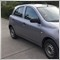# Discussion of article "Application of the Eigen-Coordinates Method to Structural Analysis of Nonextensive Statistical Distributions"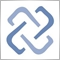Moderator
236246

The major problem of applied statistics is the problem of accepting statistical hypotheses. It was long considered impossible to be solved. The situation has changed with the emergence of the eigen-coordinates method. It is a fine and powerful tool for a structural study of a signal allowing to see more than what is possible using methods of modern applied statistics. The article focuses on practical use of this method and sets forth programs in MQL5. It also deals with the problem of function identification using as an example the distribution introduced by Hilhorst and Schehr.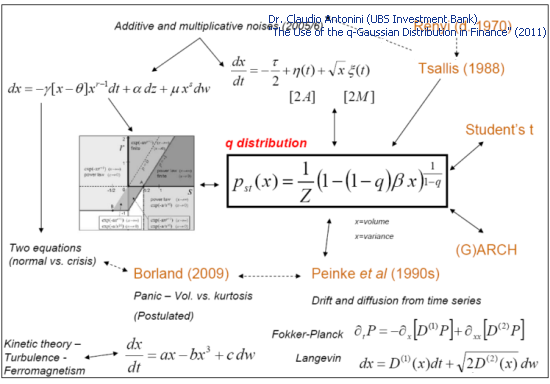Author: MetaQuotes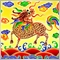23625

## 403 - запрещено. Доступ запрещен.

### Предоставленные учетные данные не дают права на просмотр этого каталога или страницы.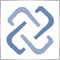2230

biantoro:

Fixed. Thank you.114

MetaQuotes,

Can you translate the discusions of the article  in Russian to English, because there are some practical applications.Google translator is no good.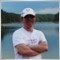17453

It is no so easy. Which one exactly do you want to be translated?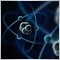472

yacoov:

MetaQuotes,

Can you translate the discusions of the article  in Russian to English, because there are some practical applications.Google translator is no good.

Let us consider the practical application of eigen-coordinates method to classical example of SP500 daily returns: (see Nonextensive Entropy: Interdisciplinary Applications)

We have used the daily data from: http://wikiposit.org/w?filter=Finance/Futures/Indices/S__and__P%20500/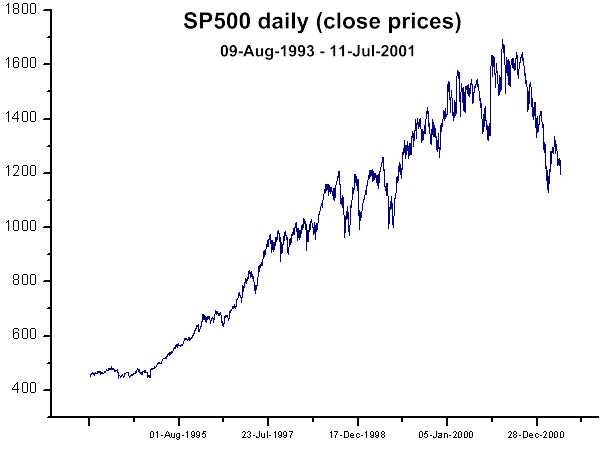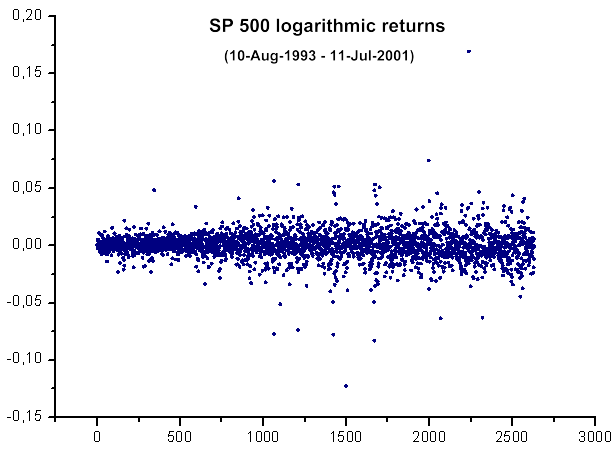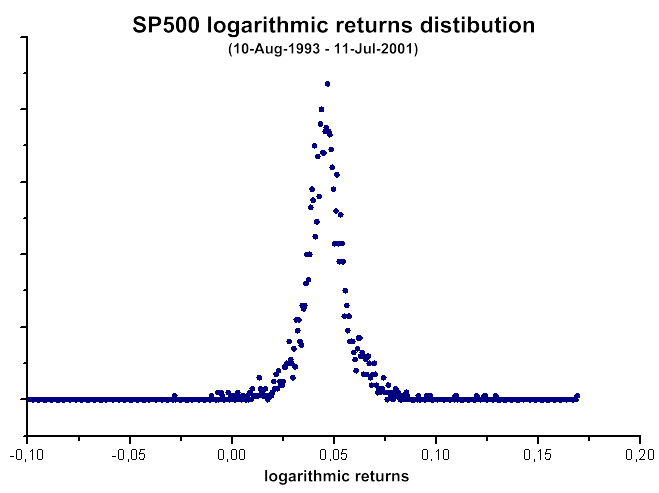To see how to perform the analysis in your terminal, the file SP500-data.csv must be placed to \Files\ folder.

After that you need to launch two scripts:

1) CalcDistr_SP500.mq5 (it calculates the distribution).

2) q-gaussian-SP500.mq5 (eigen-coordinates analysis)

The results are:

```2012.06.29 20:01:19    q-gaussian-SP500 (EURUSD,D1)    2: theta=1.770125768485269
2012.06.29 20:01:19    q-gaussian-SP500 (EURUSD,D1)    1: theta=1.864132228192338
2012.06.29 20:01:19    q-gaussian-SP500 (EURUSD,D1)    2: a=2798.166930885822
2012.06.29 20:01:19    q-gaussian-SP500 (EURUSD,D1)    1: a=8676.207867097581
2012.06.29 20:01:19    q-gaussian-SP500 (EURUSD,D1)    2: x0=0.04567518783335043
2012.06.29 20:01:19    q-gaussian-SP500 (EURUSD,D1)    1: x0=0.0512505923716428
2012.06.29 20:01:19    q-gaussian-SP500 (EURUSD,D1)    C1=-364.7131366394939
2012.06.29 20:01:19    q-gaussian-SP500 (EURUSD,D1)    C2=37.38352859698793
2012.06.29 20:01:19    q-gaussian-SP500 (EURUSD,D1)    C3=-630.3207508306047
2012.06.29 20:01:19    q-gaussian-SP500 (EURUSD,D1)    C4=28.79001868944634
2012.06.29 20:01:19    q-gaussian-SP500 (EURUSD,D1)    1  0.00177913 0.03169294 0.00089521 0.02099064 0.57597695
2012.06.29 20:01:19    q-gaussian-SP500 (EURUSD,D1)    2  0.03169294 0.59791579 0.01177430 0.28437712 11.55900584
2012.06.29 20:01:19    q-gaussian-SP500 (EURUSD,D1)    3  0.00089521 0.01177430 0.00193200 0.04269286 0.12501732
2012.06.29 20:01:19    q-gaussian-SP500 (EURUSD,D1)    4  0.02099064 0.28437712 0.04269286 0.94465120 3.26179090
2012.06.29 20:01:09    CalcDistr_SP500 (EURUSD,D1)    checking distibution cnt=2632.0 n=2632
2012.06.29 20:01:09    CalcDistr_SP500 (EURUSD,D1)    Min=-0.1229089015984444 Max=0.1690557338964631 range=0.2919646354949075 size=2632
2012.06.29 20:01:09    CalcDistr_SP500 (EURUSD,D1)    Total data=2633```

The estimated value of q, derived by eigen-coordinates method (q=1+1/theta): q~1,55

The value, reported in the book  (Fig.4 of the article) q~1.4.

Now let's check does q-gaussian look like the native function: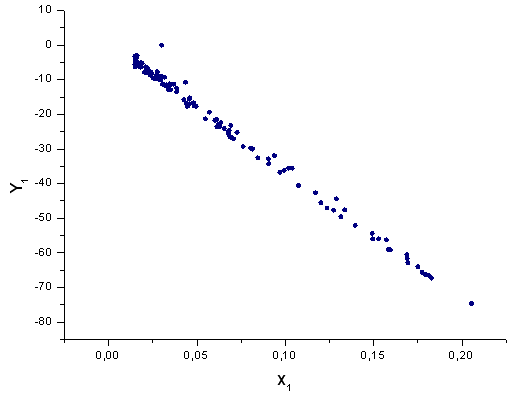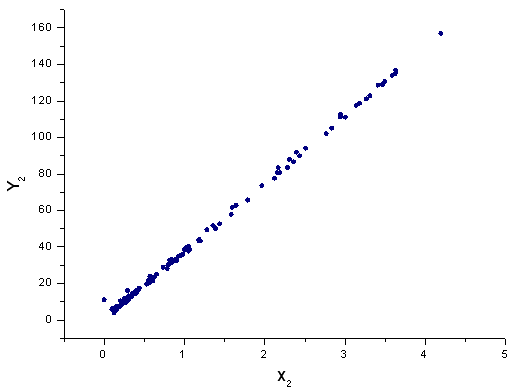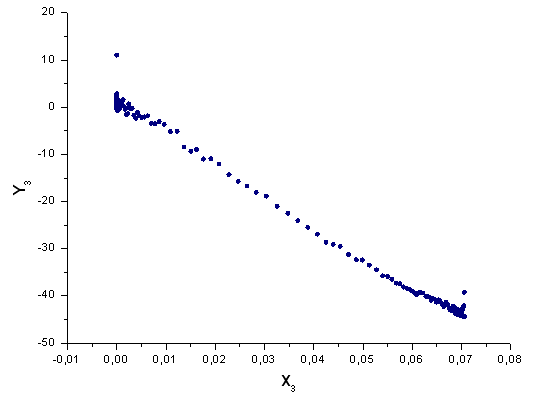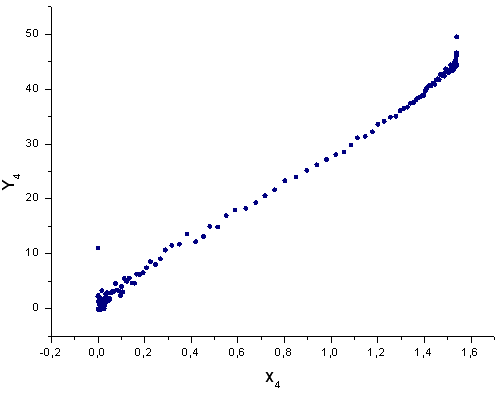Conclusions: Generally, one can see that these data can be described by q-gaussian function. It explains the successful interpretation using q-gaussian, reported in the book.

The raw ("as is") data are used, but don't forget that we deal with the "smoothed" data (indirect averaging, because the index consist of many stocks + daily data).

X1 and X2 are very sensible because of their structure, also we have the deformed tails on X3 and X4, but anyway the q-gaussian looks very close to the "native" function of the SP500 daily data returns distribution.

The shape of the X1 and X2 can be improved (linearlized) by using the integrated values (the integral form like JX1 and JX2 will lead to the straight lines).  The tails on X3 and X4 can be improved if we generalize the formula: (x-x0)^2  --> (x^2+bx+c) (but it leads to the new parameters) Similarly, the cubic case (1+a(x-x0)^3)^theta and its generalization can be considered.

Is the q-gaussian native for all financial instruments?  It's necessary to consider the instrument/timeframe dependence.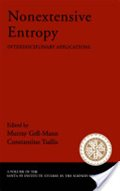Nonextensive Entropy : Interdisciplinary Applications